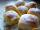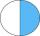Cakes

1/3 poppy cake, 1/3 apple, 15 pieces of cheese. How many are totally cakes?

Result

n =  45

Solution:

1/3 n + 1/3 n + 15 = n

n = 45

n = 45

Calculated by our simple equation calculator.

Leave us a comment of example and its solution (i.e. if it is still somewhat unclear...):Be the first to comment!To solve this example are needed these knowledge from mathematics:

Need help calculate sum, simplify or multiply fractions? Try our fraction calculator. Do you have a linear equation or system of equations and looking for its solution? Or do you have quadratic equation?

Next similar examples:

1. Jam cakesMom baked a third of plum jam cakes, one third cheesecakes and 18 poppy. How many cakes she had bake?
2. CakesOn the bowl were a few cakes. Jane ate one-third of them, Dana ate a quarter of those cakes that remained. a) What part (of the original number of cakes) Dana ate? b) At least how many cakes could be (initially) on thebowl?
3. Forest nurseryIn the forest nursery after winter, they found that 1/10 stems died out of them. For them, they land 193 new spruces. How many spruces are in the forest nursery?Mom bake cookies. Rolo took 2/9 of all cookies, Michal 3/9. How many cookies ate Rolo if Michal had 9.
5. Lengths of the poolMiguel swam 6 lengths of the pool. Mat swam 3 times as far as Miguel. Lionel swam 1/3 as far as Miguel. How many lengths did mat swim?
6. Equation 20In given equation: 8/9-4/5=2/9+x, find x
7. Simple equation 5Solve equation with fractions: X × 3/8 = 1/2
8. Eq1Solve equation: 4(a-3)=3(2a-5)
9. If-then equationIf 5x - 17 = -x + 7, then x =
10. DenominatorCalculate the missing denominator x: ?
11. Discount saleAfter the discount, the computer costs 9600, - CZK. How much did it cost when the price was reduced: a) by half b) by a third c) by one fifth and then by 160 CZKAdded together and write as decimal number: LXVII + MLXIVWrite the fraction 3/22 as a decimal.Write the mixed number as an improper fraction. 166 2/3An ant climbs 2/5 of the pole on the first hour and climbs 1/4 of the pole on the next hour. What part of the pole does the ant climb in two hours?Zdeněk picked up 15 l of water from a 100-liter full-water barrel. Write a fraction of what part of Zdeněk's water he picked.Write as a fraction and a decimal. One and two plus three and five hundredths